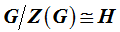#### 期刊菜单

Nonexistence of Some Finite p-Groups of the Central Quotient Order of p6
DOI: 10.12677/PM.2018.81005, PDF, HTML, XML, 下载: 1,229  浏览: 2,463  科研立项经费支持

Abstract: Based on Rodney James’ paper (The groups of order p6 (p an odd prime), Mathematics of Compu-tation, 1980, 34 (150): 613-637. ) and the idea of schreier extension theory , act extended element on extended group, by the transposition substructure and the power structure we get the contradiction of Z(G) that is cyclical group, and then we get a class finite p-groups that the central quotient are nonexistence, that is to say when H are the groups of Φ39 family of order p6 and satisfied, we get the nonexistence of G.

1. 引言

2. 基本引理

${\left(xy\right)}^{n}={x}^{n}{y}^{n}{c}_{2}^{\left(\begin{array}{c}n\\ 2\end{array}\right)}{c}_{31}^{\left(\begin{array}{c}n\\ 3\end{array}\right)}{c}_{32}^{\left(\begin{array}{c}n\\ 2\end{array}\right)+2\left(\begin{array}{c}n\\ 3\end{array}\right)}{c}_{41}^{\left(\begin{array}{c}n\\ 4\end{array}\right)}{c}_{42}^{\left(\begin{array}{c}n\\ 3\end{array}\right)+3\left(\begin{array}{c}n\\ 4\end{array}\right)}{c}_{44}^{2\left(\begin{array}{c}n\\ 3\end{array}\right)+3\left(\begin{array}{c}n\\ 4\end{array}\right)}cd$ ,

$c={c}_{51}^{\left(\begin{array}{c}n\\ 5\end{array}\right)}{c}_{52}^{3\left(\begin{array}{c}n\\ 4\end{array}\right)+4\left(\begin{array}{c}n\\ 5\end{array}\right)}{c}_{54}^{\left(\begin{array}{c}n\\ 3\end{array}\right)+6\left(\begin{array}{c}n\\ 4\end{array}\right)+6\left(\begin{array}{c}n\\ 5\end{array}\right)}{c}_{58}^{3\left(\begin{array}{c}n\\ 4\end{array}\right)+4\left(\begin{array}{c}n\\ 5\end{array}\right)}$ , $d={d}_{1}^{\left(\begin{array}{c}n\\ 3\end{array}\right)+7\left(\begin{array}{c}n\\ 4\end{array}\right)+6\left(\begin{array}{c}n\\ 5\end{array}\right)}{d}_{2}^{6\left(\begin{array}{c}n\\ 3\end{array}\right)+18\left(\begin{array}{c}n\\ 4\end{array}\right)+12\left(\begin{array}{c}n\\ 5\end{array}\right)}$ ,

$\left[{y}^{k},{x}^{n}\right]={c}_{2}^{nk}{c}_{31}^{\left(\begin{array}{c}n\\ 2\end{array}\right)k}{c}_{32}^{n\left(\begin{array}{c}k\\ 2\end{array}\right)}{c}_{41}^{\left(\begin{array}{c}n\\ 3\end{array}\right)k}{c}_{42}^{\left(\begin{array}{c}n\\ 2\end{array}\right)\left(\begin{array}{c}k\\ 2\end{array}\right)}{c}_{44}^{n\left(\begin{array}{c}k\\ 3\end{array}\right)}{c}_{51}^{\left(\begin{array}{c}n\\ 4\end{array}\right)k}{c}_{52}^{\left(\begin{array}{c}n\\ 3\end{array}\right)\left(\begin{array}{c}k\\ 2\end{array}\right)}{c}_{54}^{\left(\begin{array}{c}n\\ 2\end{array}\right)\left(\begin{array}{c}k\\ 3\end{array}\right)}{c}_{58}^{n\left(\begin{array}{c}k\\ 4\end{array}\right)}e$ ,

$e={d}_{1}^{n\left(\begin{array}{c}n\\ 2\end{array}\right)\left(\begin{array}{c}k\\ 2\end{array}\right)+\left(\begin{array}{c}n\\ 3\end{array}\right)k}{d}_{2}^{\left(\begin{array}{c}n\\ 2\end{array}\right)\left(\begin{array}{c}k\\ 2\end{array}\right)+{n}^{2}\left(\begin{array}{c}k\\ 2\end{array}\right)+2{n}^{2}\left(\begin{array}{c}k\\ 3\end{array}\right)}$ .

1) $\left[{a}^{n},b\right]={\left[a,b\right]}^{n}$

2) $\left[a,{b}^{n}\right]={\left[a,b\right]}^{n}$

3) ${\left(ab\right)}^{n}={a}^{n}{b}^{n}{\left[b,a\right]}^{\left(\begin{array}{c}n\\ 2\end{array}\right)}$

1) 若 $y\in {G}^{\prime }$ ，则 $\left[z,xy\right]=\left[z,x\right]\left[z,y\right]$$\left[xy,z\right]=\left[x,z\right]\left[y,z\right]$

2) 对 $\forall x,y,z\in G$ ，有 $\left[x,y,z\right]\left[y,z,x\right]\left[z,x,y\right]=1$

$\left[ia,jb\right]=\left[a,b,\underset{i-1}{\underset{︸}{a,\cdots ,a}},\underset{j-1}{\underset{︸}{b,\cdots ,b}}\right]$ ,

${\left(a{b}^{-1}\right)}^{m}={a}^{m}\underset{i+j\le m}{\prod }{\left[ia,jb\right]}^{\left(\begin{array}{c}m\\ i+j\end{array}\right)}{b}^{-m}$ .

3. 主要结果

${c}_{2}=\left[{\alpha }_{1}^{x},\alpha \right]={\alpha }_{1}^{-x}{\left({\alpha }_{1}{\alpha }_{2}\right)}^{x}={\alpha }_{1}^{-x}{\alpha }_{1}^{x}\underset{i+j\le p}{\prod }{\left[i{\alpha }_{1},j{\alpha }_{2}^{-1}\right]}^{\left(\begin{array}{c}p\\ i+j\end{array}\right)}{\alpha }_{2}^{x}={\alpha }_{4}^{-\left(\begin{array}{c}x\\ 2\end{array}\right)}{\left[{\alpha }_{4}^{-1},{\alpha }_{1}\right]}^{\left(\begin{array}{c}x\\ 3\end{array}\right)}{\alpha }_{2}^{x}={\alpha }_{2}^{x}{\alpha }_{4}^{-\left(\begin{array}{c}x\\ 2\end{array}\right)}{\alpha }_{5}^{-\left( x 3 \right)}$

${c}_{31}=\left[{c}_{2},\alpha \right]=\left[{\alpha }_{2}^{x}{\alpha }_{4}^{-\left(\begin{array}{c}x\\ 2\end{array}\right)}{\alpha }_{5}^{-\left(\begin{array}{c}x\\ 3\end{array}\right)},\alpha \right]=\left[{\alpha }_{2}^{x},\alpha \right]={\alpha }_{3}^{x}{\alpha }_{5}^{-\left(\begin{array}{c}x\\ 2\end{array}\right)}$ ,

${c}_{32}=\left[{c}_{2},{\alpha }_{1}^{x}\right]=\left[{\alpha }_{2}^{x}{\alpha }_{4}^{-\left(\begin{array}{c}x\\ 2\end{array}\right)}{\alpha }_{5}^{-\left(\begin{array}{c}x\\ 3\end{array}\right)},{\alpha }_{1}^{x}\right]={\left[{\alpha }_{2},{\alpha }_{1}^{x}\right]}^{x}{\alpha }_{5}^{-x\left(\begin{array}{c}x\\ 2\end{array}\right)}={\alpha }_{4}^{-{x}^{2}}{\alpha }_{5}^{-2x\left(\begin{array}{c}x\\ 2\end{array}\right)}$ ,

${c}_{41}=\left[{c}_{31},\alpha \right]=\left[{\alpha }_{3}^{x}{\alpha }_{5}^{-\left(\begin{array}{c}x\\ 2\end{array}\right)},\alpha \right]={\alpha }_{4}^{x}$ , ${c}_{42}=\left[{c}_{31},{\alpha }_{1}^{x}\right]=\left[{\alpha }_{3}^{x}{\alpha }_{5}^{-\left(\begin{array}{c}x\\ 2\end{array}\right)},{\alpha }_{1}^{x}\right]={\alpha }_{5}^{{x}^{2}}$ ,

${c}_{44}=\left[{c}_{32},{\alpha }_{1}^{x}\right]=\left[{\alpha }_{4}^{-{x}^{2}}{\alpha }_{5}^{-2x\left(\begin{array}{c}x\\ 2\end{array}\right)},{\alpha }_{1}^{x}\right]={\alpha }_{5}^{-{x}^{3}}$ , ${c}_{51}=\left[{c}_{41},\alpha \right]=\left[{\alpha }_{4}^{x},\alpha \right]=1$ ,

${c}_{52}=\left[{\alpha }_{4}^{x},{\alpha }_{1}^{x}\right]={\alpha }_{5}^{{x}^{2}}$ , ${c}_{54}=\left[{c}_{42},{\alpha }_{1}^{x}\right]=\left[{\alpha }_{5}^{{x}^{2}},{\alpha }_{1}^{x}\right]=1$ ,

${c}_{58}=\left[{c}_{44},{\alpha }_{1}^{x}\right]=\left[{\alpha }_{5}^{-{x}^{3}},{\alpha }_{1}^{x}\right]=1$ , ${d}_{1}=\left[{c}_{31},{c}_{2}\right]={\alpha }_{5}^{-{x}^{2}}$ ,

${d}_{2}=\left[{c}_{32},{c}_{2}\right]=\left[{\alpha }_{4}^{-{x}^{2}}{\alpha }_{5}^{-2x\left(\begin{array}{c}x\\ 2\end{array}\right)},{\alpha }_{2}^{x}{\alpha }_{4}^{-\left(\begin{array}{c}x\\ 2\end{array}\right)}{\alpha }_{5}^{-\left(\begin{array}{c}x\\ 3\end{array}\right)}\right]=1$ , ${\left(\alpha {\alpha }_{1}^{x}\right)}^{p}={\alpha }^{p}{\alpha }_{1}^{xp}{\alpha }_{5}^{-2{x}^{2}\left(\begin{array}{c}p\\ 5\end{array}\right)}$ .

1) 令 $H={\Phi }_{39}\left({21}^{4}\right){\text{a}}_{\text{r}}\cong G/Z\left(G\right)=〈\stackrel{¯}{\alpha },\stackrel{¯}{{\alpha }_{1}},\stackrel{¯}{{\alpha }_{2}},\stackrel{¯}{{\alpha }_{3}},\stackrel{¯}{{\alpha }_{4}},\stackrel{¯}{{\alpha }_{5}}|\left[\stackrel{¯}{{\alpha }_{i}},\stackrel{¯}{\alpha }\right]=\stackrel{¯}{{\alpha }_{i+1}}$${\left[\stackrel{¯}{{\alpha }_{4}},\stackrel{¯}{{\alpha }_{1}}\right]}^{\text{k}}={\stackrel{¯}{\alpha }}^{p}={\left[\stackrel{¯}{{\alpha }_{2}},\stackrel{¯}{{\alpha }_{3}}\right]}^{\text{k}}={\left[\stackrel{¯}{{\alpha }_{3}},\stackrel{¯}{{\alpha }_{1}}\right]}^{\text{k}}={\stackrel{¯}{{\alpha }_{5}}}^{\text{k}}$$\left[\stackrel{¯}{{\alpha }_{1}},\stackrel{¯}{{\alpha }_{2}}\right]=\stackrel{¯}{{\alpha }_{4}}$${\stackrel{¯}{{\alpha }_{1}}}^{p}={\stackrel{¯}{{\alpha }_{i+1}}}^{p}={\stackrel{¯}{{\alpha }_{5}}}^{p}=\stackrel{¯}{1}\left(i=1,2,3\right)〉$ ，则有 ${\left(\alpha {\alpha }_{1}^{x}\right)}^{p}={\alpha }_{5}^{\text{k}-2{x}^{2}\left(\begin{array}{c}p\\ 5\end{array}\right)}$ 。如果 $p>5$ ，则不存在群 $G$ 使得 $G/Z\left(G\right)\cong H$ 。此时若假设 $p=5$ ，因为 $\text{k}-2{x}^{2}\equiv 0$ 至多有两个根，所以存在 $x$$1\le x\le 4$ 使得 $\text{k}-2{x}^{2}\overline{)\equiv }0$ 。因为 $H=〈\alpha ,\alpha {\alpha }_{1}^{x}〉$ ，所以不存在群 $G$ 使得 $G/Z\left(G\right)\cong H$

2) 令 $H={\Phi }_{39}\left({21}^{4}\right){\text{b}}_{\text{r}}\cong G/Z\left(G\right)=〈\stackrel{¯}{\alpha },\stackrel{¯}{{\alpha }_{1}},\stackrel{¯}{{\alpha }_{2}},\stackrel{¯}{{\alpha }_{3}},\stackrel{¯}{{\alpha }_{4}},\stackrel{¯}{{\alpha }_{5}}|\left[\stackrel{¯}{{\alpha }_{i}},\stackrel{¯}{\alpha }\right]=\stackrel{¯}{{\alpha }_{i+1}}$${\left[\stackrel{¯}{{\alpha }_{4}},\stackrel{¯}{{\alpha }_{1}}\right]}^{\text{r}}={\stackrel{¯}{\alpha }}^{p}={\left[\stackrel{¯}{{\alpha }_{2}},\stackrel{¯}{{\alpha }_{3}}\right]}^{\text{r}}={\stackrel{¯}{{\alpha }_{1}}}^{p}={\stackrel{¯}{{\alpha }_{5}}}^{\text{r}}$$\left[\stackrel{¯}{{\alpha }_{1}},\stackrel{¯}{{\alpha }_{2}}\right]=\stackrel{¯}{{\alpha }_{4}}$ , ${\stackrel{¯}{{\alpha }_{i+1}}}^{p}={\stackrel{¯}{{\alpha }_{5}}}^{p}=\stackrel{¯}{1}\left(i=1,2,3\right)〉$ ，则 ${\left(\alpha {\alpha }_{1}^{x}\right)}^{p}={\alpha }_{5}^{\text{r}+\text{r}x-2{x}^{2}\left(\begin{array}{c}p\\ 5\end{array}\right)}$ 。如果 $p>5$ ，则不存在群 $G$ 使得 $G/Z\left(G\right)\cong H$ 。此时假设 $p=5$ ，则 ${\left(\alpha {\alpha }_{1}^{x}\right)}^{5}={\alpha }_{5}^{\text{r}+\text{r}x-2{x}^{2}}$ 。因为 $\text{r}+\text{r}x-2{x}^{2}\equiv 0$ 至多有两个根，所以存在 $x$$1\le x\le 4$ 使得 $\text{r}+\text{r}x-2{x}^{2}\overline{)\equiv }0$ 。因为 $H=〈\alpha ,\alpha {\alpha }_{1}^{x}〉$ ，所以不存在群 $G$ 使得 $G/Z\left(G\right)\cong H$

3) 令 $H={\Phi }_{39}\left({21}^{4}\right){\text{b}}_{\text{p}+\text{r}}\cong G/Z\left(G\right)=〈\stackrel{¯}{\alpha },\stackrel{¯}{{\alpha }_{1}},\stackrel{¯}{{\alpha }_{2}},\stackrel{¯}{{\alpha }_{3}},\stackrel{¯}{{\alpha }_{4}},\stackrel{¯}{{\alpha }_{5}}|\left[\stackrel{¯}{{\alpha }_{i}},\stackrel{¯}{\alpha }\right]=\stackrel{¯}{{\alpha }_{i+1}}$$\left[\stackrel{¯}{{\alpha }_{1}},\stackrel{¯}{{\alpha }_{2}}\right]=\stackrel{¯}{{\alpha }_{4}}$ ,

${\left[\stackrel{¯}{{\alpha }_{2}},\stackrel{¯}{{\alpha }_{3}}\right]}^{\text{k}}={\left[\stackrel{¯}{{\alpha }_{3}},\stackrel{¯}{{\alpha }_{1}}\right]}^{\text{k}}={\left[\stackrel{¯}{{\alpha }_{4}},\stackrel{¯}{{\alpha }_{1}}\right]}^{\text{k}}={\stackrel{¯}{{\alpha }_{1}}}^{p}={\stackrel{¯}{{\alpha }_{5}}}^{\text{k}}$${\stackrel{¯}{\alpha }}^{p}={\stackrel{¯}{{\alpha }_{i+1}}}^{p}={\stackrel{¯}{{\alpha }_{5}}}^{p}=\stackrel{¯}{1}\text{\hspace{0.17em}}\left(i=1,2,3\right)〉$ ，则 ${\left(\alpha {\alpha }_{1}^{x}\right)}^{p}={\alpha }_{5}^{\text{k}x-2{x}^{2}\left(\begin{array}{c}p\\ 5\end{array}\right)}$ 。如果 $p>5$ ，则不存在群 $G$ 使得 $G/Z\left(G\right)\cong H$ 。此时假设 $p=5$ ，则 ${\left(\alpha {\alpha }_{1}^{x}\right)}^{5}={\alpha }_{5}^{\text{k}x-2{x}^{2}}$ 。因为 $\text{k}x-2{x}^{2}\equiv 0$ 至多有两个根，所以存在 $x$ 使 $\text{k}x-2{x}^{2}\overline{)\equiv }0$ 。因为 $〈{\alpha }_{1},\alpha {\alpha }_{1}^{x}〉=H$ ，所以不存在群 $G$ 使得 $G/Z\left(G\right)\cong H$

4) 令 $H={\Phi }_{39}\left({1}^{6}\right)\cong G/Z\left(G\right)=〈\stackrel{¯}{\alpha },\stackrel{¯}{{\alpha }_{1}},\stackrel{¯}{{\alpha }_{2}},\stackrel{¯}{{\alpha }_{3}},\stackrel{¯}{{\alpha }_{4}},\stackrel{¯}{{\alpha }_{5}}|\left[\stackrel{¯}{{\alpha }_{i}},\stackrel{¯}{\alpha }\right]=\stackrel{¯}{{\alpha }_{i+1}}$$\left[\stackrel{¯}{{\alpha }_{1}},\stackrel{¯}{{\alpha }_{2}}\right]=\stackrel{¯}{{\alpha }_{4}}$${\stackrel{¯}{{\alpha }_{1}}}^{p}={\stackrel{¯}{\alpha }}^{p}={\stackrel{¯}{{\alpha }_{i+1}}}^{p}={\stackrel{¯}{{\alpha }_{5}}}^{p}=\stackrel{¯}{1}$$\left[\stackrel{¯}{{\alpha }_{2}},\stackrel{¯}{{\alpha }_{3}}\right]=\left[\stackrel{¯}{{\alpha }_{3}},\stackrel{¯}{{\alpha }_{1}}\right]=\left[\stackrel{¯}{{\alpha }_{4}},\stackrel{¯}{{\alpha }_{1}}\right]=\stackrel{¯}{{\alpha }_{5}}$$\left(i=1,2,3\right)〉$ ，则 ${\left(\alpha {\alpha }_{1}^{x}\right)}^{5}={\alpha }_{5}^{-2{x}^{2}}$ 。因为 $H=〈\alpha {\alpha }_{1},\alpha {\alpha }_{1}^{2}〉$ ，如果 $p=5$ ，则不存在群 $G$ 使得 $G/Z\left(G\right)\cong H$ 。此时假设 $p>5$ 。不妨设 $\left[{\alpha }_{i},\alpha \right]={\alpha }_{i+1}$$\left[{\alpha }_{4},{\alpha }_{1}\right]={\alpha }_{5}$ ，由于 $\left[{\alpha }_{i},\alpha \right]={\alpha }_{i+1}a{}_{i}$$\left[{\alpha }_{4},{\alpha }_{1}\right]={\alpha }_{5}b$${a}_{i},b\in Z\left(G\right)$ ，令 ${{\alpha }^{\prime }}_{i+1}={\alpha }_{i+1}{a}_{i}$${{\alpha }^{\prime }}_{5}={\alpha }_{5}b$ ，则 ${\alpha }_{i+1}={{\alpha }^{\prime }}_{i+1}{a}_{i}{}^{-1}$${\alpha }_{5}={{\alpha }^{\prime }}_{5}{b}^{-1}$ 。此时 $\left[\alpha ,{\alpha }_{i+3}\right]$$\left[{\alpha }_{1},{\alpha }_{5}\right]$$\left[{\alpha }_{4},{\alpha }_{5}\right]$$\left[{\alpha }_{2},{\alpha }_{i+3}\right]$$\left[{\alpha }_{3},{\alpha }_{i+3}\right]\in Z\left(G\right)$ 。 因为 $\left[{\alpha }_{5},{\alpha }_{i}\right]={\left[{\alpha }_{5},{\alpha }_{i}\right]}^{\alpha }=\left[{\alpha }_{5},{\alpha }_{i}{\alpha }_{i+1}\right]=\left[{\alpha }_{5},{\alpha }_{i+1}\right]\left[{\alpha }_{5},{\alpha }_{i}\right]$ ，所以 $\left[{\alpha }_{5},{\alpha }_{i+1}\right]=1$ 。 因为 $\left[{\alpha }_{2},{\alpha }_{4}\right]={\left[{\alpha }_{2},{\alpha }_{4}\right]}^{\alpha }=\left[{\alpha }_{2}^{\alpha },{\alpha }_{4}^{\alpha }\right]=\left[{\alpha }_{2}{\alpha }_{3},{\alpha }_{4}\right]=\left[{\alpha }_{2},{\alpha }_{4}\right]\left[{\alpha }_{3},{\alpha }_{4}\right]$ ，所以 $\left[{\alpha }_{3},{\alpha }_{4}\right]=1$ 。 因为 ${\alpha }_{5}^{\alpha }=\left[{\alpha }_{4}^{\alpha },{\alpha }_{1}^{\alpha }\right]=\left[{\alpha }_{4},{\alpha }_{1}{\alpha }_{2}\right]=\left[{\alpha }_{4},{\alpha }_{2}\right]{\alpha }_{5}$ ，所以 $\left[{\alpha }_{5},\alpha \right]=\left[{\alpha }_{4},{\alpha }_{2}\right]$ 。 因为 $\left[{\alpha }_{3},{\alpha }_{1}\right]={\left[{\alpha }_{3},{\alpha }_{1}\right]}^{{\alpha }_{2}}=\left[{\alpha }_{3}^{{\alpha }_{2}},{\alpha }_{1}^{{\alpha }_{2}}\right]=\left[{\alpha }_{3}{\alpha }_{5}^{-1},{\alpha }_{1}{\alpha }_{4}\right]=\left[{\alpha }_{3},{\alpha }_{1}\right]\left[{\alpha }_{5}^{-1},{\alpha }_{1}\right]$ ，所以 $\left[{\alpha }_{5},{\alpha }_{1}\right]=1$ 。令 $\left[{\alpha }_{2},{\alpha }_{3}\right]={\alpha }_{5}z$ ，其中 $z\in Z\left(G\right)$ ，则因为有 ${\alpha }_{5}\left[{\alpha }_{5},\alpha \right]={\alpha }_{5}^{\alpha }={\left[{\alpha }_{2},{\alpha }_{3}\right]}^{\alpha }{z}^{-1}=\left[{\alpha }_{2}{\alpha }_{3},{\alpha }_{3}{\alpha }_{4}\right]{z}^{-1}=\left[{\alpha }_{2},{\alpha }_{4}\right]\left[{\alpha }_{2},{\alpha }_{3}\right]{z}^{-1}=\left[{\alpha }_{2},{\alpha }_{4}\right]{\alpha }_{5}$ ，所以 $\left[{\alpha }_{5},\alpha \right]=\left[{\alpha }_{2},{\alpha }_{4}\right]$ ，这意味着 $\left[{\alpha }_{5},\alpha \right]=1$ 。又因为 $G=〈\alpha ,{\alpha }_{1},Z\left(G\right)〉$${\alpha }_{5}\in Z\left(G\right)$ ，矛盾，所以不存在群 $G$ 使得 $G/Z\left(G\right)\cong H$ 。至此，定理证明完毕。

  James, R. (1980) The Groups of Order p6 (p an Odd Prime). Mathematics of Computation, 34, 613-637.  Lan, Y.H., Hui, M., Ban, G.N. and Shao, M.W. (2012) The Order of the Automorphism Groups of all Groups of Oder p5. Chinese Quarterly Journal of Mathematics, 27, 495-503.  惠敏. p6阶若干家族群的自同构群的阶[J]. 宝鸡文理学院学报(自然科学版), 2016, 36(3): 1-7.  Davitt. R.M. (1980) On the Automorphism Group of a Finite p-Group with a Small Central Quotient. Canadian Journal of Mathematics, 32, 1168-1176.  Ban, G.N., Zhang, J.S. and Yu, S.X. (1996) The Lower Bound for the Order of the Automorphism Groups. Proceedings of the Royal Irish Academy, 96, 159-167.  班桂宁, 崔艳, 刘海林. 中心循环且中心商群的阶为p6的一类新LA-群[J]. 广西师范学院学报(自然科学版), 2014, 31(2): 1-3.  班桂宁, 刘海林, 崔艳. 中心循环且中心商的阶为p6的LA-群[J]. 重庆理工大学学报(自然科学版), 2014, 28(1): 120-122.  班桂宁, 许永峰, 陈倩, 赵丽萍. 一类中心商同构于第四十家族的LA-群[J]. 贵州大学学报(自然科学版), 2015, 32(1): 1-4.  班桂宁, 聂婷婷, 陈科成. p6阶 家族群的扩张[J]. 湖州师范学院学报(自然科学版), 2015, 37(10): 1-8.  班桂宁, 田甜, 王玉琪. 基于p6阶第三十家族群的新LA-群[J]. 井冈山大学学报(自然科学版), 2015, 36(5): 14-20.  陈科成, 班桂宁, 聂婷婷. 中心商同构于p6阶第十九家族的一类LA-群[J]. 浙江科技学院学报(自然科学版), 2016, 28(5): 337-343.  马玉龙, 伍星, 刘海林. 一类新LA-群的研究[J]. 钦州学院学报(自然科学版), 2017, 32(7): 20-23.  班桂宁, 俞曙霞. 具有循环中心和小中心商的有限p-群[J]. 广西大学学报(自然科学版), 1993, 18(3): 15-22.  徐明曜. 有限群导引(上,下) [M]. 第2版. 北京: 科学出版社, 2001.